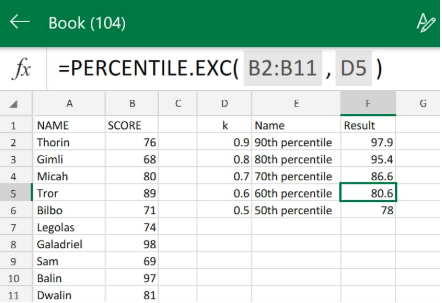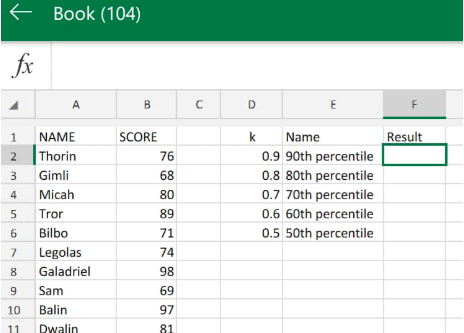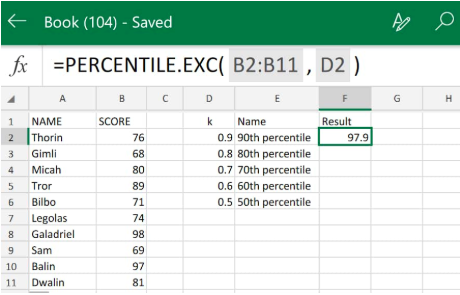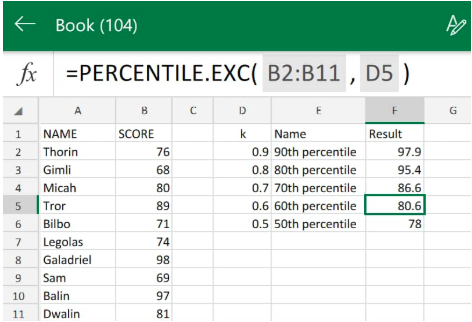Get instant live expert help with Excel or Google Sheets“My Excelchat expert helped me in less than 20 minutes, saving me what would have been 5 hours of work!”

#### Post your problem and you’ll get expert help in seconds.

Your message must be at least 40 characters
Our professional experts are available now. Your privacy is guaranteed.

# Percentile function in Excel – Excelchat

The Percentile Calculator in Excel returns the “k-th percentile” from a supplied data set.

We can use Percentile exc function to calculate Excel 90th percentile rank, the 70th percentile rank, etc.Figure 1. Percentile Function in Excel

## Generic Formula

`=PERCENTILE.EXC(array, k)`

• Array = A set of data values
• k – A number between 0 and 1 representing the k:th percentile

A percentile is simply a value under which a specified percentage of data set values fall.

The Excel Percentile function is an built-in operation which is categorized under Excel’s Statistical Functions.

## How to Calculate Percentiles in Excel

We will now show you how to find percentiles in Excel by using the following simple steps;

1. Let’s say we are required to analyze the total number of students who ranked above a specified percentile in an entrance exam. We begin by collecting the data available to us inside our Excel worksheet;Figure 2. of Percentile Data in Excel

Be sure to allocate a column of  empty cell for Percentiles exc to return our resulting number values (column F above.

1. The Percentile rank formula, which we will enter into cell F2 of our worksheet example is shown below:
`=PERCENTILE.EXC(B2:B11,D2)`Figure 3. Percentile Function in Excel

The Percentile exc function returned 97.9 as the Excel 90th percentile rank value for the array of scores in column B of our worksheet example.

1. Copy the modified version of the Percentile rank formula down into the other empty cells in column F to obtain the expected percentile ranking results;Figure 4. of Percentiles in Excel

## Note

A percentile that is calculated with a k value of 4 means that 40% percent of its values are below or equal to its calculated result, while  a percentile that is calculated with a k value of.9 means that 90% percent of its values are below or equal to its calculated result.

## Instant Connection to an Excel Expert

Most of the time, the problem you will need to solve will be more complex than a simple application of a formula or function. If you want to save hours of research and frustration, try our live Excelchat service! Our Excel Experts are available 24/7 to answer any Excel question you may have. We guarantee a connection within 30 seconds and a customized solution within 20 minutes.